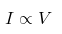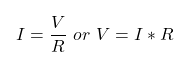# What is Ohm’s Law

## Ohm’s Law

Ohm’s Law is a basic principle in electrical engineering  which describes the relationship between three fundamental electrical quantities: voltage (V), current (I), and resistance (R).

It is named after the German physicist Georg Simon Ohm, who formulated this law in the early 19th century.

### What is Ohm’s Law ?

According to Ohm’s Law the electrical current(I) flowing through any conductor is directly proportional to the potential difference (voltage)(V) between its ends, assuming the physical conditions (e.g. temperature) of the conductor remain constant.

### Mathematical Expression of Ohm’s Law

Mathematically Ohm’s Law is expressed as:Introducing the constant of proportionality, the resistance R in the above equation, we get,Where:

• V (Voltage) is measured in volts (V).
• I (Current) is measured in amperes (A).
• R (Resistance) is measured in ohms (Ω).

Here’s an explanation of each of these components:

1. Voltage (V): Voltage is the electrical potential difference between two points in an electrical circuit. It represents the force that pushes electric charge (electrons) through a conductor (such as a wire).
2. Current (I): Current is the flow of electric charge through a conductor. It represents the rate of flow of electrons in a circuit. Current is measured in amperes (A).
3. Resistance (R): Resistance is a property of a material or component that opposes the flow of current. It’s a measure of how difficult it is for electrons to pass through a conductor. Resistance is measured in ohms (Ω).

Ohm’s Law essentially states that in an ideal, linear electrical circuit, the voltage across a resistor (or any component with resistance) is directly proportional to the current passing through it, and the constant of proportionality is the resistance of the component. In other words:

• If you increase the voltage (V) across a component while keeping the resistance (R) constant, the current (I) through the component will increase.

V ↑ = I ↑* R (Constant)

• If you increase the resistance (R) of a component while keeping the voltage (V) constant, the current (I) through the component will decrease.

V (Constant) = I ↓* R ↑

• If you know any two of the three values (V, I, and R), you can use Ohm’s Law to calculate the third value.

Ohm’s Law is a foundational principle in electronics and is used extensively in the design and analysis of electrical circuits, helping engineers and scientists understand and predict how voltage, current, and resistance relate to one another in various electrical components and systems.